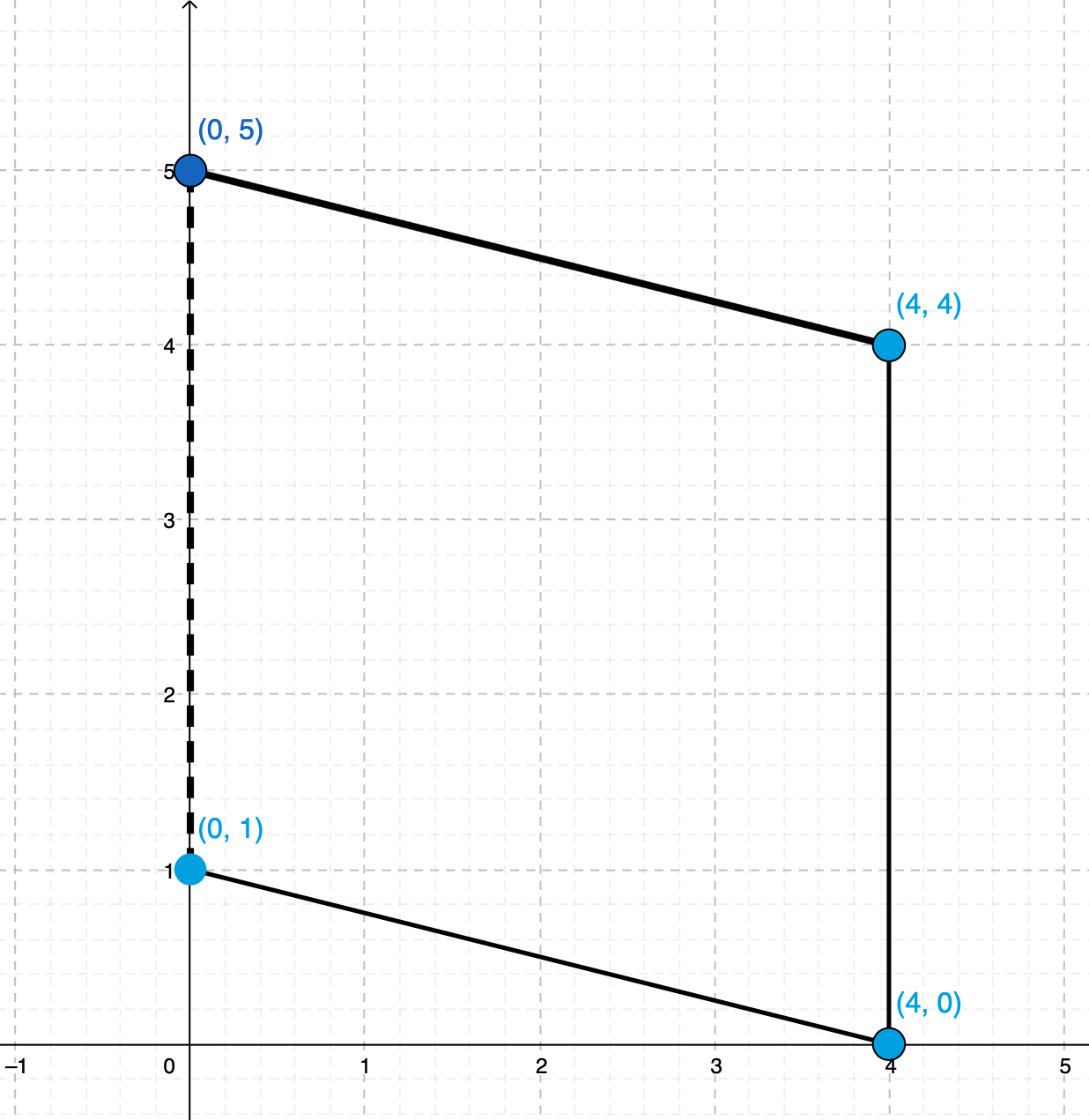# Find the fourth vertex of the rectangle

2. In the xy-coordinate plane, the coordinates of three vertices of a parallelogram are (4, 4), (4, 0), and (0, 1). What are the coordinates of the fourth vertex of the parallelogram?

1. (0, 5)
2. (0, 4)
3. (0, 3)
4. (4, 1)
5. (2, 6)

We can plot the three given points on xy-plane. Since the left side of the parallelogram must have same length as the right side (top and bottom sides must also be equals) so the forth point must be (0, 5), see the following figure: